# AP Statistics : How to identify characteristics of a normal distribution

## Example Questions

### Example Question #1 : How To Identify Characteristics Of A Normal Distribution

Which parameters define the normal distribution?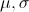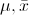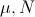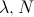Explanation:

The two main parameters of the normal distribution are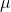and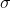.is a location parameter which determines the location of the peak of the normal distribution on the real number line.is a scale parameter which determines the concentration of the density around the mean. Larger's lead the normal to spread out more than smaller's.

### Example Question #2 : How To Identify Characteristics Of A Normal Distribution

All normal distributions can be described by two parameters: the mean and the variance. Which parameter determines the location of the distribution on the real number line?

Both

Standard Deviation

Variance

Mean

Mean

Explanation:

The mean determines where the normal distribution lies on the real number line, while the variance determines the spread of the distribution.

### Example Question #11 : Normal Distribution

Which of the following populations has a precisely normal distribution?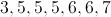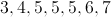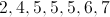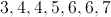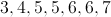Explanation:

A normal distribution is one in which the values are evenly distributed both above and below the mean.  A population has a precisely normal distribution if the mean, mode, and median are all equal.  For the population of 3,4,5,5,5,6,7, the mean, mode, and median are all 5.

### Example Question #12 : Normal Distribution

If a population has a normal distribution, the number of values within one positive standard deviation of the mean will be . . .

equal to the number of values within two negative standard deviations of the mean

fewer than 20% of all values in the population

at least 40% of all values in the population

fewer than the number of values within one negative standard deviation of the mean

equal to the number of values within one negative standard deviation of the mean

equal to the number of values within one negative standard deviation of the mean

Explanation:

In a normal distribution, the number of values within one positive standard deviation of the mean is equal to the number of values within one negative standard deviation of the mean.  The reason for this is that the values below the population mean exactly parallel the values above the mean.

### Example Question #13 : Normal Distribution

Consider a normal distribution with a mean ofand a standard deviation of. Which of the following statements are true according to the Empirical Rule?

1.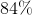of observations are at least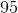.
2.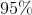of observations are betweenand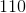.
3.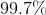of observations are betweenand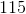.

2 only

2 and 3

3 only

1 only

1, 2 and 3

1, 2 and 3

Explanation:

2) and 3) are true by definition of the Empirical Rule - also known as the 68-95-99.7 Rule. Using our information with mean of 100 and a standard deviation of 5 we can create a bell curve with 100 in the middle. One standard deviation out from the mean would give us a range from 95 to 105 and would be in our 68% section. If we go two standard deviations out from 100 we would get the range 90 to 110 thus lying in the 95% section. Lastly, when we go out 3 standard deviations we get a range of 85 to 115 thus falling within the 99.7% section.

1) can be deduced as true because it means that 68% of observations are between 95 and 105, and removing one of those bounds (namely, the upper one) adds the 16%, since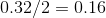, of observations larger than 105 leading to 68 + 16 = 84% of observations greater than 95.

### Example Question #14 : Normal Distribution

What is the relationship between the mean and the median in a normally distributed population?

The mean and median should be the same value.

The mean is larger than the median.

You can not measure these pieces of information in a normal distribution.

The median is larger than the mean.

The mean and median should automatically be zero.

The mean and median should be the same value.

Explanation:

A normal distrubtion is completely symmetrical and has not outliers.  This means that the mean is not pulled to either side by outliers and should lie directly in the middle along with the median (the middle number of the distribution).

### Example Question #15 : Normal Distribution

Which is the following is NOT a property of the normal distribution?

The probability that a random data point is within one standard deviation of the mean is approximately 78%

The normal curve is symmetrical about the mean μ

The total area under the curve is equal to 1

The mean is at the middle and divides the area into halves

The probability that a random data point is within one standard deviation of the mean is approximately 78%

Explanation:

The empirical rule tells us that the probability that a random data point is within one standard deviation of the mean is approximately 68%, not 78%.

### Example Question #16 : Normal Distribution

Which is the following is true about the standard normal distribution?

It has a mean of 0 and a standard deviation of 1

It has a mean of 0 and a standard deviation of 0

It has a mean of 1 and a standard deviation of 0

It has a mean of 1 and a standard deviation of 1

It has a mean of 0 and a standard deviation of 1

Explanation:

The standard normal distribution is just like any other normal distribution that you might have looked at except that it has a standard deviation of 1 and a mean of 0.

### Example Question #17 : Normal Distribution

Cheyenne is worried about food thieves in the break room at work, and she believes that, as the week progresses, and people get lazy and ready for the weekend, more food theft occurs. She gathered the following data on number of thefts per day, and fell very behind in her work for a week.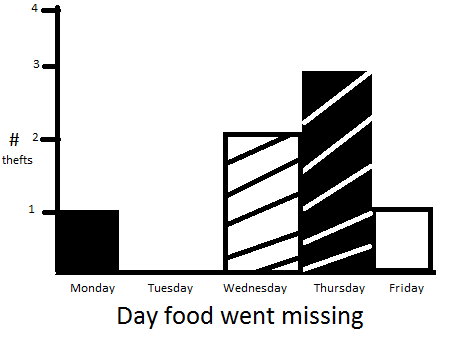Which of the following statements about the data are true?

i: the data is normally distributed

ii: the data is skewed left

iii: the data supports Cheyenne's theory

iv: the data is a representative sample

ii, iii & iv

i, ii, and iii

i, iii & iv

iii only

ii & iii only

ii & iii only

Explanation:

The data is not normal by virtue of being skewed left, which also supports Cheyenne's theory... there is no way of knowing wether this data was a representative sample, but also no option with ii, iii and iv was provided to avoid frustration/confusion

### Example Question #18 : Normal Distribution

In order to be considered a normal distribution, a data set (when graphed) must follow a bell-shaped symmetrical curve centered around the mean.

It must also adhere to the empirical rule that indicates the percentage of the data set that falls within (plus or minus) 1, 2 and 3 standard deviations of the mean.

In order to be a normal distribution, what percentage of the data set must fall within:

1)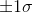2)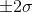3)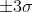1) 68

2) 95

3) 98.7

1) 65

2) 98

3) 99.7

1) 65

2) 98

3) 99.9

1) 68

2) 95

3) 98

1) 68

2) 95

3) 99.7

1) 68

2) 95

3) 99.7

Explanation:

1) Percentile for Z=1 is .8413 - or - .1587 in one tail - or - .3174 in both tails -

1 - .3174=.6826

2) Percentile for Z=2 is .9772 - or - .0228 in one tail - or - .0456 in both tails -

1 - .0456=.9544

3) Percentile for Z=3 is .9987 - or - .0013 in one tail - or - .0026 in both tails -

1 - .0026=.9974